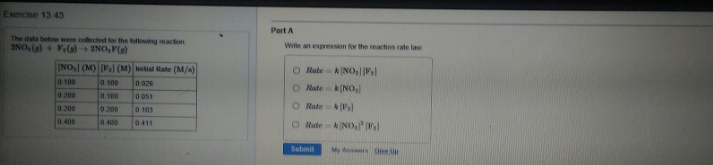# Problem: The data below were collected for the following reaction 2NO2 (g) + F2 (g) → 2NO2F (g) Part AWrite an expression for the reaction rate law. (i) Rate = k[NO3][F2] (ii) Rate = k[NO2] (iii) Rate = k[F2] (iv) Rate = k[NO3]2 [F2]

🤓 Based on our data, we think this question is relevant for Professor Mignerey's class at UMD.

###### FREE Expert Solution###### Problem Details

The data below were collected for the following reaction

2NO2 (g) + F2 (g) → 2NO2F (g)

Part A

Write an expression for the reaction rate law.

(i) Rate = k[NO3][F2

(ii) Rate = k[NO2

(iii) Rate = k[F2

(iv) Rate = k[NO3][F2]